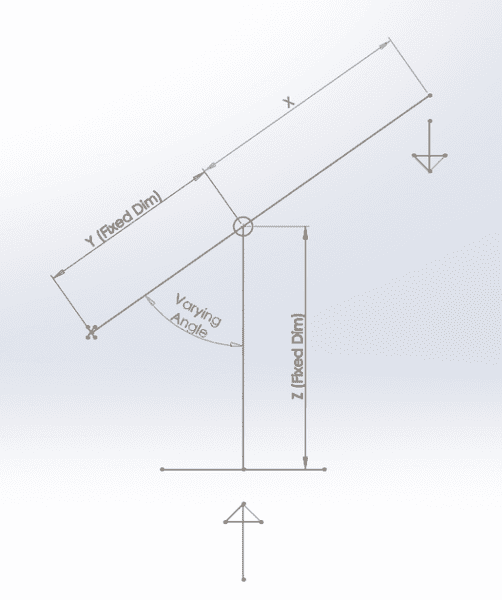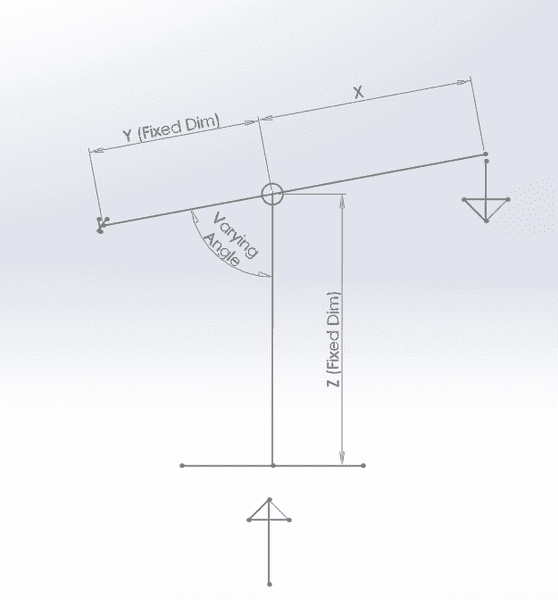User Name Remember Me? Password

 Physics Physics Forum - General Physics Discussion and Physics NewsFeb 27th 2017, 02:29 AM #1 Junior Member   Join Date: Nov 2016 Posts: 4 Leverage and Forces on a Pivot System The diagram drawn below shows a lever system fixed to a point on the left with a pivot point (circle) on the line which attaches to vertical line. I have dimensions for Y and Z and the forces pulling the lever down on the right, and the force acting upwards from below. I am trying to work out the dimension for X to determine how long the lever needs to be to compress the upwards force, but I am unsure due to the varying angle as the lever is pulled down. Any help is greatly appreciated.Before lever is pulled down.After lever is pulled down. Thanks. Tags: cold future maroon 5 lyrics, closer halsey the chainsmokers lyrics, black beatles gucci mane rae sremmurd lyrics Last edited by adamjackson; Feb 28th 2017 at 12:02 AM.Feb 27th 2017, 06:37 AM #2 Physics Team   Join Date: Jun 2010 Location: Morristown, NJ USA Posts: 2,354 Your sketch is difficult to understand. You say there is a "fixed point" at the left (which would be a pivot) plus a pivot at the circle. How can a lever have two pivot points? If the lever rotates about the fixed point at the left, then the dimension Z will compress as the lever rotates, yet you call Z a "fixed dimension" - so clearly I don't understand the configuration. So I'll give two answers - maybe one of them captures your intent: 1. If the fixed pivot is at the left end (where the "X" is), so that Z compresses as the lever rotates, then if you calculate the torque about the left end of the lever and set it to zero you get: $\displaystyle F_d (x+y) = F_uy$ where F_u is the upward force and F_d is the downward force applied at the right end of the lever. Rearranging: $\displaystyle F_d = F_u \frac y {x+y}$ This is independent of the angle, as long as both forces act purely in the vertical direction. Rearrange to find x: $\displaystyle x = \frac {(F_u-F_d)y}{F_d}$ If x is greater than or equal to this value the lever will rotate clockwise. If less than this value it will rotate counterclockwise. 2. If the fixed pivot is at the point where the upward force acts, and the left end has no forces acting on it and is free to rise, then zero force is required at the right end of the lever in order to rotate it clockwise, and it doesn't matter what the length of "X" is, as long as it's greater than 0. Perhaps you can clarify what the point "X" is meant to signify, what Z is, and where the upward force comes from as well. topsquark likes this. Last edited by ChipB; Feb 27th 2017 at 12:19 PM.Tags forces, leverage, pivot, systemThread ToolsShow Printable VersionEmail this Page Display ModesLinear ModeSwitch to Hybrid ModeSwitch to Threaded ModeSimilar Physics Forum Discussions Thread Thread Starter Forum Replies Last Post mrb11 Equilibrium and Elasticity 6 Jun 7th 2013 02:15 PM Caiti Kinematics and Dynamics 2 Nov 30th 2010 01:00 PM kingman Kinematics and Dynamics 3 Jun 28th 2010 11:33 AM Apprentice123 Kinematics and Dynamics 0 Apr 16th 2009 04:25 PM Apprentice123 Kinematics and Dynamics 2 Apr 12th 2009 09:51 AM